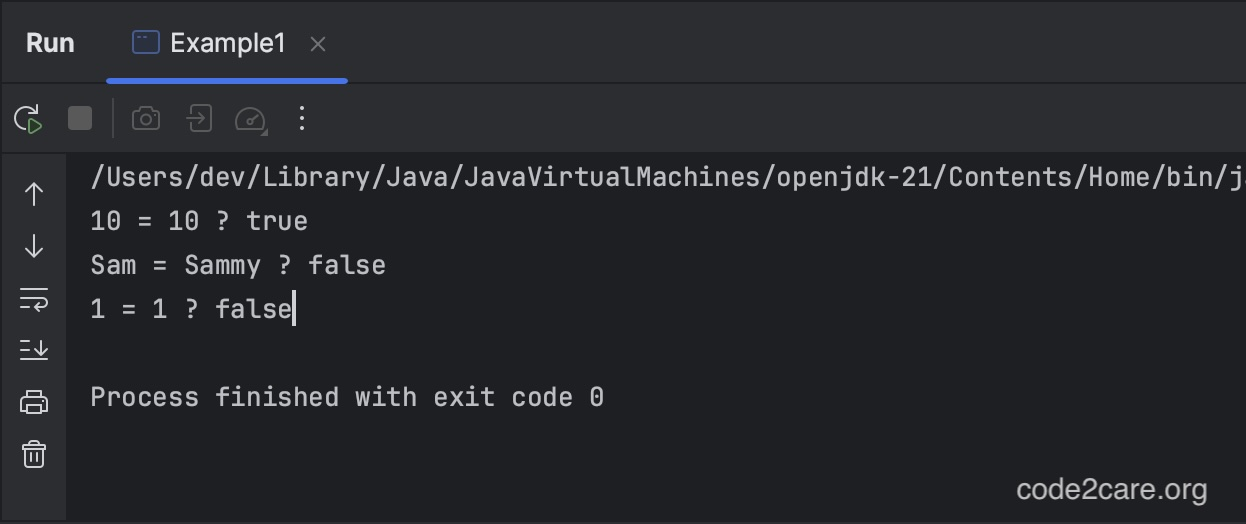# Java Generics Methods Examples

In Java Generics, like Generic Classes and Interfaces we can have Generic Methods with their own `type parameters`.

Note: The Generic Methods type parameter's scope is limited to the method where it is declared.

## Example 1: Compare two values

``````public class Example1 {

public <T> boolean areEqual(T value1, T value2) {
return value1.equals(value2);
}

public static void main(String[] args) {

Example1 example = new Example1();

int num1 = 10;
int num2 = 10;
boolean isEqual1 = example.areEqual(num1, num2);
System.out.println(num1 + " = " + num2 + " ? " + isEqual1);

String str1 = "Sam";
String str2 = "Sammy";
boolean isEqual2 = example.areEqual(str1, str2);
System.out.println(str1 + " = " + str2 + " ? " + isEqual2);

String val1 = "1";
int val2 = 1;
boolean isEqual3 = example.areEqual(val1, val2);
System.out.println(val1 + " = " + val2 + " ? " + isEqual3);

}
}``````
Output:## Example 2: Find First Occurrence of an Element in an Array

``````import java.util.List;

public class Example2 {

public <T> int findIndex(T[] array, T target) {
for (int i = 0; i < array.length; i++) {
if (array[i].equals(target)) {
return i;
}
}
}

public <T> int countOccurrences(List<T> list, T target) {
int count = 0;
for (T item : list) {
if (item.equals(target)) {
count++;
}
}
return count;
}

public static void main(String[] args) {
Example2 example = new Example2();

Integer[] intArray1 = { 1, 2, 3, 4, 5 };
int index1 = example.findIndex(intArray1, 3);
System.out.println("Index of 3 in the array is: " + index1);

String[] strArray = { "China", "Japan", "India", "USA", "Canada" };
int index2 = example.findIndex(strArray, "USA");
System.out.println("Index of 'USA' in the array is: " + index2);

Integer[] intArray2 = { 5, 6, 2, 4, 6, 7, 8, 1, 9 };
int index3 = example.findIndex(intArray2, 17);
System.out.println("Index of 17 in the array is: " + index3);

}
}``````
Output:

Index of 3 in the array is: 2
Index of 'USA' in the array is: 3
Index of 17 in the array is: -1

## Example 3: Count Occurrences of an Element in a List

``````import java.util.Arrays;
import java.util.List;

public class Example3 {

public <T> int countOccurrences(List<T> list, T target) {
int count = 0;
for (T item : list) {
if (item.equals(target)) {
count++;
}
}
return count;
}

public static void main(String[] args) {
Example3 example = new Example3();

List<String> names = Arrays.asList("Japan", "China", "Japan", "Australia", "India", "Japan");
int count1 = example.countOccurrences(names, "Japan");
System.out.println("Occurrences of 'Japan' in the list: " + count1);

List<Integer> numbers = Arrays.asList(3, 4, 6, 9, 3);
int count2 = example.countOccurrences(numbers, 3);
System.out.println("Occurrences of '3' in the list: " + count2);
}
}``````
Output:

Occurrences of 'Japan' in the list: 3
Occurrences of '3' in the list: 2

-

### Facing issues? Have Questions? Post them here! I am happy to answer!

Author Info:

Rakesh (He/Him) is a seasoned developer with over 10 years of experience in web and app development, and a deep knowledge of operating systems. Author of insightful How-To articles for Code2care.